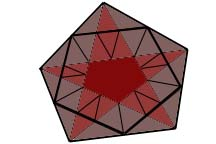This document was released at 5:00 PM on 1/30/99

### Areas of Midpoint Polygons

The midpoint polygon of a polygon is the polygon formed by the midpoints of the edges, taken in the same order. What is the relationship between the area of the midpoint polygon and the area of the original polygon? Suggest (and prove?) generalizations.

• "MP" at 6:29 PM on 1/29/99

As demonstrated in class, the midpoint polygon (MP) of a triangle is a second triangle similar to the first, with 1/4 the area.

Also shown in class was the fact that the MP of a convex quadrilateral is a parallelogram. By similar argument it can be shown that the MP of any planar quadrilateral is a parallelogram (I haven't looked at non-planar figures yet). This can be seen by constructing the diagonals of the original figure and using the fact that the base of any triangle is parallel to the line containing the midpoints of the opposite sides.

Conjectured in class was that the area of a quadrilateral's MP is 1/2 the original (oriented) area. I haven't tried it with nonconvex figures yet, but it is true for convex quadrilaterals:

The diagonals of the original quadrilateral divide it into four triangles. Consider one of these. The region enclosed by both this triangle and the MP is a parallelogram. The two areas not in the MP are congruent triangles (play around with it). The parallelogram can be divided into two triangles congruent to these outer triangles. Thus, the area of the MP within each of the four main triangles is 1/2 the area of each triangle. And finally, the area of the MP is half the area of the original quadrilateral.

Now on to nonconvex quadrilaterals, pentagons, and non-planar polygons...

• "Professor" at 7:19 PM on 1/29/99

Your proof or the 1/2 conjecture for convex quadrilaterals is very convincing. It will be interesting to see how it works for the non-convex and self-intersecting quadrilaterals. And what about pentagons? And other possible generalizations? Good start.

• "MP" at 3:32 PM on 1/30/99

More on the 1/2 area conjecture.

For non-convex (but non-self-intersecting) quadrilaterals the argument is similar to the one for convex quadrilaterals. In such a figure there is one angle that is greater than 180° (in a sense). Extend the diagonal that contains this vertex so it divides the original quadrilateral into two triangles and the MP into two parallelograms. Each of these triangles has a corresponding parallelogram which is half its area (the parallelogram's base is half the triangle's base and its height is half the triangle's height). And there it is.

The self-intersecting case is a bit harder to explain. The conjecture is that the area of the MP is 1/2 the difference of the areas of the two lobes of the original. Let the original quadrilateral be ABCD, with AD and BC intersecting at O. Let the midpoint of AB be E, BC be F, etc., so EFGH forms the MP. Now extend DB indefinitely and extend FE to intersect DB at I and GH to intersect DB at J.

The area of the new parallelogram FGJI is 1/2 the area of the triangle BCD. Also, the area of the smaller parallelogram EHJI is 1/2 the area of the triangle ABD. Since
a(COD) - a(AOB) = a(CBD) - a(ADB) and
a(FGJI) - a(EHJI) = a(EFGH),
The area of MP EFGH is 1/2 the difference of the areas of triangles AOB and COD. QED.

Of course, you really have to draw it out to see what's happening.

• "Professor" at 4:29 PM on 1/30/99

I agree that a diagram would help--why not look at some other entries and find a picture you would like to use as a model and learn how to use the relevant program, assuming that it is available? Let me know. Also, do you need to consider some special cases? You extend a pair of segments at some stage so that their lines intersect. What if they are parallel, or is that somehow ruled out?

• "MP" at 8:19 PM on 1/31/99

A diagram to go along with the explanation above: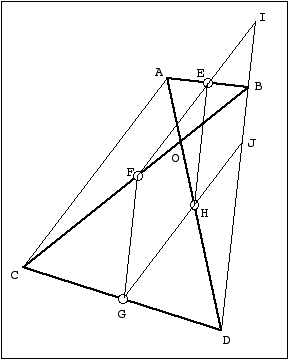By the way, when the diagonals are parallel (this means that the oriented area of the quadrilateral is zero) it is impossible to extend the sides of the MP to a diagonal. But this is OK, because the area of the MP in this case is zero.

• "Professor" at 112:27 AM on 2/1/99

Very nice indeed. What program did you use? Does it compute areas for you as well as draw the picture? Does it know when the two lines are parallel?

• "SK" at 3:29 PM on 1/30/99

I looked at two things regarding this topic.

The first thing I did was find a general formula for the area of a midpoint polygon when working with regular polygons.

I did this by calculating the area of a regular x-gon with sides length n. I broke the x-gon down into 2x right triangles, each with short side n/2, and was able to figure out a general formula for area.

Then I figured out the area of the "discard triangles" from the outside of the midpoint polygon. This was a similar task, and again, there are 2x of them.

With some trig, I found that the area of the midpoint polygon was:

(2xn^2 cot (180/x) - xn^2 sin (180(x-2)/2))/8

The ratio is easy to figure out now, because we already know that the first half of that expression is the area of the polygon.

Kind of messy, but at least a formula.

----

The second thing I began to look at was area ratios of polygons made by third-points, or fourth-points...instead of midpoints.

Clearly the limit of the area as each of the midpoints is moved toward the next point (counter- or) clockwise is the area of the polygon. But at what rate does it approach this value?

So far, I've only investigated squares:

If the side of the square is length n and we break the side into n/x and (x-n)/x, the area of the inner square is:

n^2(x^2 -2x + 2)/x^2

So with midpoints, we get (1/2)n^2, as expected.
With third points, it's (5/9)n^2,
quarter points is (5/8)n^2
fifth points is (17/25)n^2

More to come...

• "JS" at 4:35 PM on 1/30/99

Ah! That's a really interesting generalisation (I don't know about your trigonometry but if you look at the preceding contribution you'll find that if your first result is right it should simplify a bit). To get back to the generalization: I think it shouldn't be too hard to find a general formula for a square of length 1 (don't bother with that n; use the n for the number of pieces your cutting the sides into). If you look a little at the fractions you've already got a pattern:

2/4 (= 1/2)
5/9
10/16 (= 5/8)
17/25

You see that the denominators are all squares, and that the numerators increase by 3, 5, 7: the odd numbers. It shouldn't be hard to find a general formula. Looking forward to hearing more.

• "JL" at 3:50 PM on 1/30/99

We established in class that for a triangle, the midpoint polygon has 1/4 the area of the original triangle. We also conjectured that for a quadrilateral, the corresponding area is 1/2, and we gave an argument for convex quadrilaterals. I will give an argument that works for any non-self-intersecting quadrilateral, whether or not it is convex.

Label our quadrilateral ABCD. If ABCD is not convex, label it such that the exterior angle less than 180° is at vertex C. Then we can draw diagonal AC which is in the interior of ABCD. Now call the midpoints E, F, G, and H as shown below. We saw in class that EF, AC, and GH are parallel, and that in fact EFGH is a parallelogram. Call L the intersection of EH and AC, and K the intersecion of FG and AC. (It may be necessary to extend line AC outside the quadrilateral.)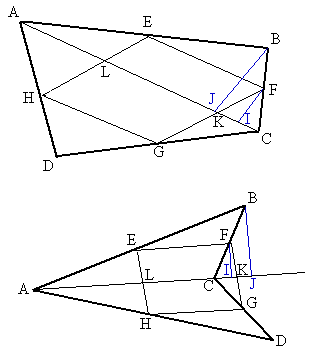Now drop a perpendicular from B to AC, intersecting AC at J. Similarly draw FI perpendicular to AC. Triangles CFI and CBJ are similar with CB = 2 CF, so BJ = 2 FI. And ABC and EBF are similar, so AC = 2 EF. Therefore, Area(ABC) = (1/2)(AC)(BJ) = (1/2)(2 EF)(2 FI) = 2 (EF)(FI) = 2 Area(EFLK), as FI is a height of parallelogram EFLK.

By the same argument, Area(ADC) = 2 Area(HGLK), so that Area(ABCD) = 2 Area(EFGH), Q.E.D.

Now we will show that no such general formula exists for n-gons with n > 4. Consider a pentagon (say) with four consecutive vertices collinear. (So the pentagon is "actually" a triangle.)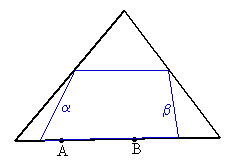It should be clear without a formal argument that by moving points A and B along their line, sides a and b can be moved to change the area of the blue midpoint polygon without changing the area of the black polygon. This argument also works for more than five sides, but not for fewer.

• "Assistant" at 4:44 PM on 1/30/99

Good results, and nice drawings. That's a crucial obersvation about the pentagon: there's no single fraction that gives the ratio of the midpoint pentagon to the outer pentagon. But that does not mean that ratio can go completely wild. Can you bracket it with a max and a min? Can you give conjectures for a max and a min? What about an n-gon?

• "JL" at 5:35 PM on 1/30/99

Well, for a hexagon, we can make the area of the midpoint polygon approach 100% of the hexagon's area by letting D approach A, E approach B, and F approach C in the diagram below.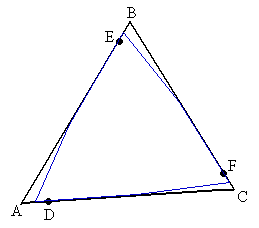This works for more than six sides also. I would conjecture that for a pentagon, the best we can do is a similar trick in which only two sides of the triangle are subdivided, with which we can approach 3/4 of the pentagon's area: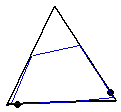Of course this doesn't address minima, only maxima. But it does give a very simple progression: for three sides, the maximum ratio is 1/4; for four sides, 2/4; for five sides, 3/4; for more than five sides, 4/4.

• "Assistant" at 1:38 PM on 1/31/99

Nice observations about the hexagon case. It seems true that we make the ratio 1 for a figure with six sides or more. Could we have the ratio greater than 1? If not, why not?

I have a hunch, too, that 3/4 isn't the max for the pentagon, though it's better than the regular pentagon ratio of 0.65 something. Work on it;)

• "JL" at 3:36 PM on 1/31/99

Ah, ha! I've found a way to get an infinite ratio for a pentagon! Earlier, I was just considering convex polygons, for which the M.P. obviously must be contained in the original polygon since the sides of the M.P. are lines between points on the polygon. But consider this bizarre, highly non-convex pentagon: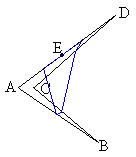I want point C to actually coincide with point A; I have just drawn them separately to make the diagram clearer. Thus, the original pentagon has zero area, but the M.P. still has positive area. So for a pentagon (or any n-gon with n > 4), there is no upper limit on the ratio of areas unless we require that the polygon be convex.

• "Professor" at 4:09 PM on 1/31/99

That's a fine initial observation and an even finer follow-up. It is important to distinguish the convex from the non-convex case, as you point out so forcefully, since in the convex case, the midpoint figure is contained in the original, therefore with area not greater than the original.
I like your conjecture about the maximum possible for a pentagon. Can you complete the proof that this will be the best? And what about the minimum ratio?

What about the length estimate by the way? Is the midpoint polygon of a convex polygon automatically convex?

With respect to non-convex polygons, your argument is striking. It is impressive to have a polygon with area zero and non-zero area midpoint polygon. Good work. Ist there anything more to be said about this area problems? What about extensions of the idea to higher dimensions?

• "SC" at 4:25 PM on 1/31/99

Here's a bit of scheme code(don't ask why - I happen to like the language) to calculate the ratios:

area of planar n-gon/area of midpoint n-gon
perimiter " " " " "/perimiter " " " " " "

If the area or perimiter of the midpoint n-gon is 0, it returns the list consisting of the area of the n-gon and 0.

the main function calls look like this:

(area-ratio 'list-of-ordered-pairs)
(perimiter-ratio 'list-of-ordered-pairs)

where the ordered pairs are the points of the n-gon, represented as something like this:
((0 0) (1 3) (2 6) .... )

Scheme Code

• "SC" at 7:21 PM on 1/31/99

In case anyone would like to find the areas using a program not written in scheme, here's the algorithm to find oriented area of a planar n-gon (including self-intersecting cases):

1) Begin with a set of verticies, (v0, v1 ... vn)
2) Apply a translation to the plane that identifies one of the verticies, v0, with the origin.
3) Create an accumulator for the area.
4) For each pair of verticies, (a b) = (v1 v2)...(vn-1 vn), add the quantity:

(axby - aybx) / 2

to the accumulator. (where ax is the x-coordinate of point a)
5) The final value of the accumulator is the oriented area of the n-gon

• "Professor" at 12:04 AM on 2/4/99

Good work. We really do need to have some formulas here in order to make headway on the general problem. By the way, does your formulation give a solution to the general quadrilateral problem? (Compare the synthetic solution by "MP".)

• "SC" at 5:35 PM on 1/31/99

The oriented area of the midpoint quadrilateral is 1/2 the oriented area of the original planar quadrilateral.

Proof:
First, observe that the oriented area of a general planar quadrilateral with verticies at (0,0), (a1,a2), (b1,b2)
, and (c1,c2) is given by the formula:

(1) A(Q) = a1b2-a2b1+b1c2-b2c1

Now, we find the positions of the midpoints, w,x,y,z:

w = (a1/2,a2/2)
x = ((a1+b1)/2,(a2+b2)/2)
y = ((b1+c1)/2,(b2+c2)/2)
z = (c1/2,c2/2)

Next, apply the translation carrying w to the origin, leaving area intact:

w -> w' = (0,0)
x -> x' = (b1/2,b2/2)
y -> y' = ((b1+c1-a1)/2,(b2+c2-a2)/2)
z -> z' = ((c1-a1)/2,(c2-a2)/2)

Applying formula (1):

A(M(Q)) = x'1y'2-x'2y'1+y'1z'2-y'2z'1

We substitute into this equation, do some algebra (which is trivial, so I'll omit it to save space) and get the final nice form:

A(M(Q)) = (a1b2-a2b1+b1c2-b2c1)/2

or,

2*A(M(Q)) = A(Q)

• "Assistant" at 11:37 PM on 1/31/99

Excellent well. Nice to see a neat algebraic solution amidst all this geometry. If you want more to work on, we're still looking to find: 1) upper bound for the area ratio in *convex* polygons of five sides or more 2) lower bound for the area ratio in polygons of five sides or more. Hope to hear from you.

• "Professor" at 12:07 AM on 2/4/99

That certainly answers the question I posed at the end of your previous entry. Perhaps I should read all the submissions before responding to any of them! I wonder if you can reformulate this in vector form, without using the subscripts. You will need to use the assumption that the quadrilateral is planar at some point though.

• "SC" at 4:44 PM on 2/4/99

I'll go one step better - I'll give the general formula for the area ratio in planar midpoint polygons:

Suppose our polygon (embedded in R2) has verticies given by the vectors {v1, v2, ... , vn}. Then the midpoint polygon has verticies given by:

u1 = (1/2)(v1 + v2)
u2 = (1/2)(v2 + v3)
...
un-1 = (1/2)(vn-1 + vn)
un = (1/2)(vn + v1)

Also, notice that using this representation for the polygons, oriented area is given by the formula:

A = (1/2)[D(v1,v2) + D(v2,v3) + ... + D(vn-1,vn) + D(vn,v1)]

Then the area of our midpoint polygon is simply:

A(M) = (1/2)[D((1/2)(v1+v2),(1/2)(v2+v3)) + ... +

D((1/2)(vn-1+vn),(1/2)(vn+v1))]

Using the properties of determinants (from good 'ol math 54), this is just:

A(M) = (1/4)[D(v1,v2)+D(v2,v3)+...+D(vn,v1)] +
(1/8)[D(v1,v3)+D(v2,v4)+...+D(vn,v2)]

Or:

A(M) = (1/2)A + (1/8)[D(v1,v3)+D(v2,v4)+...+D(vn,v2)]

So what is the second term? It corresponds to one-quarter of the area(s) of the related polgon(s) created by taking the points in the following order for odd n:

{v1,v3,v5,...,vn,v2,v4,...,vn-1}

and for even n:

{v1,v3,v5,...,vn-1}
{v2,v4,v6...,vn}

And, in a wonderful fit of consistency, this agrees with what we've already observed for quadrilaterals and regular n-gons.

• "Professor" at 10:04 PM on 2/4/99

This is really exciting, especially the geometric interpretation of the "second term". The computation comes out really nicely if you employ complex numbers. If you consider (a-bi)(c+di) = ac + bd +i(ad-bc), then we can write the oriented area of the triangle determined by the origin, a+bi and c+di as (1/2)Im (a+bi)(c+di), where the underlining stands for complex conjugation. Then the oriented area of a polygon with vertices z1,z2,...,zn will be (1/2) IM (Sum zjzj+1 = A(P).

We then can apply this to find the oriented area of the midpoint polygon as follows: A(M(P)) = (1/2)Im Sum (1/4)(zj-1+zj)(zjzj+1) = (1/2)A(P) + (1/8) Im Sum zjzj + (1/8) Im Sum zj-1zj+1. Since zjzj is real for all j, one of these sums equals 0 and the other, as you very nicely observe, is one-fourth the oriented area of the "star polygon" obtained by taking the odd terms in order followed by the even terms. This is particularly nice in the case of the pentagon.

• "Assistant" at 8:48 PM on 3/14/99

Here's a late comment. This is basically to give an intuitional explanation for the formula you obtained, namely that

A(MP)=A(P)*(1/2)+A(SP)*(1/4)

Where A() is the area function, MP is the midpoint polygon, SP is the starshaped polygon with the area at center counted twice.

If we take a plogon with the starshaped polygon drawn inside it: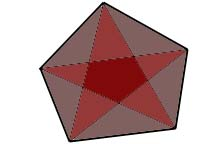Then there are three kinds of areas: the grey triangles that touch an edge of the original polygon, the pinkish triangles that don't touch an edge but that touch a vertex, and the center region. We can write the area of the star-shaped polygon as {0,1,2} where the three numbers are coefficients that mutliply the area of different colors, the first number multiplying the grey area, the second number the pinkish area, the third number the central region (that way the central region is counted twice). Similarly, the area of the original polygon can be written {1,1,1}. The identity translates to:

A(MP)={1,1,1}*(1/2)+{0,1,2}*(1/4)={1/2,3/4,1}

And behold: